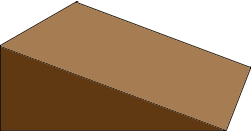SEARCH HOMEMath Central Quandaries & QueriesQuestion from David: I have small equipment hut that has a small attic space that I want to blow insulation into it. The attic size is shaped like a triangle that is about 8 feet by 10 feet by 7 inches at the highest point. I need to estimate the volume in square feet or cubic feet to know how many bags of insulation to blow into the space. ThanksHi David,

I think the attic looks something like the diagram below.If so the volume is $\large \frac12 \normalsize 10 \times 8 \times \large \frac{7}{12} \normalsize = 23 \large \frac13 \normalsize \mbox{ cubic feet.}$

If I have the incorrect shape write back, preferably with a diagram.

PennyMath Central is supported by the University of Regina and The Pacific Institute for the Mathematical Sciences.